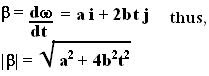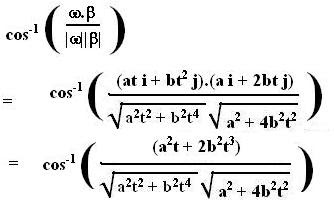## Saturday, August 4, 2007

### Irodov Problem 1.56

(a) The magnitude of the angular velocity vector is given by the Euclidean norm as,. The angular acceleration is the time-derivative of the angular velocity vector and is given by,(b) The angle between the vector is given by,